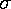## ANOVA: ANalysis Of VAriance between groups

You might guess that the size of maple leaves depends on the location of the trees. For example, that maple leaves under the shade of tall oaks are smaller than the maple leaves from trees in the prairie and that maple leaves from trees in median strips of parking lots are smaller still. To test this hypothesis you collect several (say 7) groups of 10 maple leaves from different locations. Group A is from under the shade of tall oaks; group B is from the prairie; group C from median strips of parking lots, etc. Most likely you would find that the groups are broadly similar, for example, the range between the smallest and the largest leaves of group A probably includes a large fraction of the leaves in each group. Of course, in detail, each group is probably different: has slightly different highs, lows, and hence it is likely that each group has a different average (mean) size. Can we take this difference in average size as evidence that the groups in fact are different (and perhaps that location causes that difference)? Note that even if there is not a "real" effect of location on leaf-size (the null hypothesis), the groups are likely to have different average leaf-sizes. The likely range of variation of the averages if our location-effect hypothesis is wrong, and the null hypothesis is correct, is given by the standard deviation of the estimated means:/N½

whereis the standard deviation of the size of all the leaves and N (10 in our example) is the number of leaves in a group. Thus if we treat the collection of the 7 group means as data and find the standard deviation of those means and it is "significantly" larger than the above, we have evidence that the null hypothesis is not correct and instead location has an effect. This is to say that if some (or several) group's average leaf-size is "unusually" large or small, it is unlikely to be just "chance".

The comparison between the actual variation of the group averages and that expected from the above formula is is expressed in terms of the F ratio:

F=(found variation of the group averages)/(expected variation of the group averages)

Thus if the null hypothesis is correct we expect F to be about 1, whereas "large" F indicates a location effect. How big should F be before we reject the null hypothesis? P reports the significance level.

In terms of the details of the ANOVA test, note that the number of degrees of freedom ("d.f.") for the numerator (found variation of group averages) is one less than the number of groups (6); the number of degrees of freedom for the denominator (so called "error" or variation within groups or expected variation) is the total number of leaves minus the total number of groups (63). The F ratio can be computed from the ratio of the mean sum of squared deviations of each group's mean from the overall mean [weighted by the size of the group] ("Mean Square" for "between") and the mean sum of the squared deviations of each item from that item's group mean ("Mean Square" for "error"). In the previous sentence mean means dividing the total "Sum of Squares" by the number of degrees of freedom.

### Why not just use the t-test?

The t-test tells us if the variation between two groups is "significant". Why not just do t-tests for all the pairs of locations, thus finding, for example, that leaves from median strips are significantly smaller than leaves from the prairie, whereas shade/prairie and shade/median strips are not significantly different. Multiple t-tests are not the answer because as the number of groups grows, the number of needed pair comparisons grows quickly. For 7 groups there are 21 pairs. If we test 21 pairs we should not be surprised to observe things that happen only 5% of the time. Thus in 21 pairings, a P=.05 for one pair cannot be considered significant. ANOVA puts all the data into one number (F) and gives us one P for the null hypothesis.